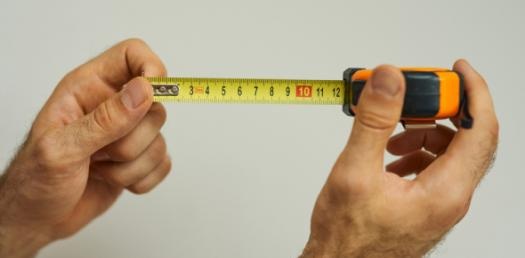# Measure Of Central Tendency

Approved & Edited by ProProfs Editorial Team
At ProProfs Quizzes, our dedicated in-house team of experts takes pride in their work. With a sharp eye for detail, they meticulously review each quiz. This ensures that every quiz, taken by over 100 million users, meets our standards of accuracy, clarity, and engagement.
| Written by Jicrozie
J
Jicrozie
Community Contributor
Quizzes Created: 1 | Total Attempts: 1,165
Questions: 5 | Attempts: 1,165Settings• 1.

### Find the mean, median, mode, and range of the data set.3, 5, 8, 3, 4, 2, 3

• A.

Mean: 4, Median: 4, Mode: 3, Range: 5

• B.

Mean: 4, Median: 3, Mode: 3, Range: 5

• C.

Mean: 4.5, Median:3, Mode: 7, Range: 5

• D.

Mean: 5, Median: 3, Mode: 3, Range: 5

B. Mean: 4, Median: 3, Mode: 3, Range: 5
Explanation
The mean is calculated by finding the sum of all the numbers in the data set and dividing it by the total number of values. In this case, the sum of 3, 5, 8, 3, 4, 2, and 3 is 28. Dividing 28 by 7 (the total number of values) gives a mean of 4. The median is the middle value when the data set is arranged in ascending order. Arranging the numbers in ascending order gives 2, 3, 3, 3, 4, 5, and 8. The middle value is 3, so the median is 3. The mode is the number that appears most frequently in the data set. In this case, 3 appears three times, which is more than any other number, so the mode is 3. The range is the difference between the largest and smallest values in the data set. The largest value is 8 and the smallest value is 2, so the range is 8 - 2 = 6. Therefore, the correct answer is Mean: 4, Median: 3, Mode: 3, Range: 5.

Rate this question:

• 2.

### Wes has kept a daily record of his phone text messages for several days. Which data set has a mode of 18?

• A.

13, 15, 17, 17, 19, 21, 23, 23

• B.

5, 7, 6, 18, 20, 18, 23, 25

• C.

20, 21, 7, 16, 15, 18, 2, 9

• D.

21, 4, 7, 9, 4, 20, 5, 3, 18

B. 5, 7, 6, 18, 20, 18, 23, 25
Explanation
The data set 5, 7, 6, 18, 20, 18, 23, 25 has a mode of 18. The mode is the value that appears most frequently in a data set. In this case, the number 18 appears twice, which is more than any other number in the set. Therefore, 18 is the mode of this data set.

Rate this question:

• 3.

### Officer Jackson is researching recorded speeds on a particular roadway. Which data set has amean closest to 34 mph?

• A.

34, 34, 36, 37, 38, 44, 45

• B.

29, 28, 31, 34, 39, 40, 50

• C.

30, 29, 28, 40, 55, 24, 25

• D.

14, 34, 25, 36, 38, 39, 54

D. 14, 34, 25, 36, 38, 39, 54
Explanation
The data set with a mean closest to 34 mph is 14, 34, 25, 36, 38, 39, 54.

Rate this question:

• 4.

### A quiz is given to 33 students. Scores on the quiz can range from 1 to 10. Based on the results below, which conclusion can be drawn regarding the measures of central tendency?1, 1, 5, 5, 5, 5, 5, 5, 6, 6, 6, 6, 6, 6, 6, 7, 7, 7, 7, 7, 7, 7, 8, 8, 8, 8, 8, 8, 8, 8, 9, 9, 9

• A.

The median is lower than the mean or mode.

• B.

The mode is lower than the mean or median.

• C.

The mean is lower than the mode or median.

• D.

The mean, medihttp://www.proprofs.com/quiz-school/submit2.phpan, and mode are approximately equal.

C. The mean is lower than the mode or median.
Explanation
The given data set is not symmetrical, as there are more occurrences of the numbers 5, 6, 7, and 8 compared to the other numbers. The mode represents the most frequently occurring value, which in this case is 5. The median is the middle value when the data set is arranged in ascending order, which is also 5. However, the mean is calculated by summing up all the values and dividing by the total number of values. Since there are more occurrences of the numbers 5, 6, 7, and 8, the mean is pulled towards these higher values, making it higher than the mode and median. Therefore, the correct conclusion is that the mean is lower than the mode or median.

Rate this question:

• 5.

### The number of boats going through a strait was recorded during 10 days as follows:36, 41, 58, 45, 36, 39, 52, 40, 43, 47Suppose the 47 should be 94 instead. Which measure of central tendency would change?

• A.

Mean

• B.

Median

• C.

Mode

• D.

All of the aboveBack to top